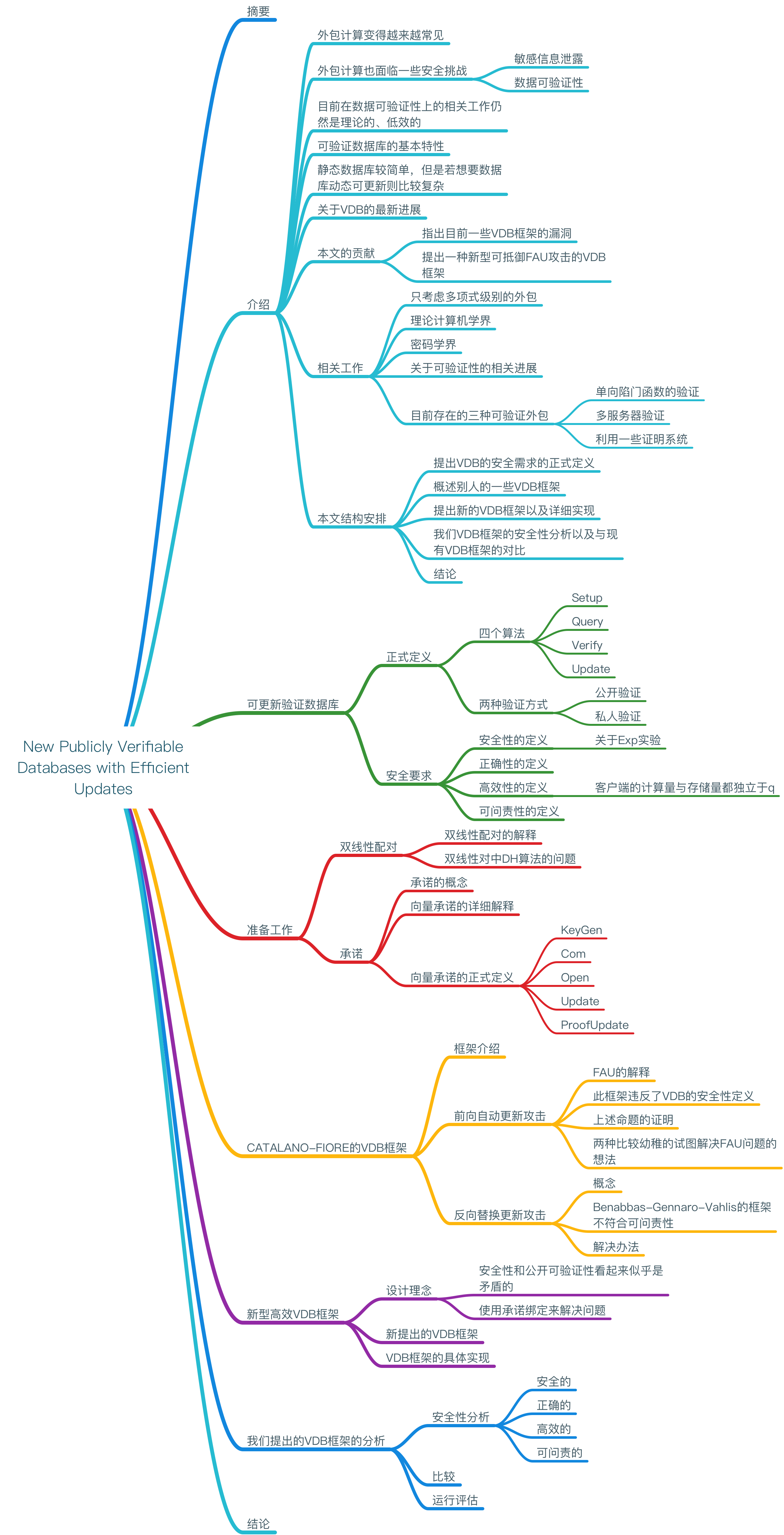## 一、引入

### 2. 文章结构

1. 提出VDB的安全要求的正式定义；
2. 概述目前已有的一些VDB框架；
3. 提出新的VDB框架及其详细实现；
4. 本文的VDB框架的安全性分析以及与现有VDB框架的对比；
5. 结论


## 二、可更新验证数据库

### VDB的主要四个算法

#### 1. 数据库初始化算法：$Setup(1^k,DB)$

Setup算法用于数据库的初始建立阶段，主要功能是由输入的安全参数$k$来产生数据库$DB$的服务端和客户端所需要用到的公钥和私钥以及数据库的编码格式$S$

#### 2. 数据查询算法：$Query(PK,S,x)$

Query算法实现的是客户端对服务器请求第$x$个数据的功能，服务器会返回一个二元组$\tau =(v,\pi)$，这里的$\pi$可以暂时理解为是对这里的密文数据$v$的一个验证。

#### 4. 数据更新算法：$Update(SK,x,v')$

$x$ 数据序号 $m_x$ 数据库中第x个数据
$v_x$ 第x个数据对应的密文 $k$ 数据库安全参数
$DB$ 数据库DB $S$ 数据库编码格式
$\tau$ $\tau =(v,\pi)$为服务器对于客户端查询请求的响应 $\pi$ 对于查询结果$v$附带的验证码
$v'$ 用于更新替代原数据$v$的新数据 $t'_x$ 用于更新第x个数据所需要的票据

## 三、新VDB框架所用到的概念

### 双线性配对

$\mathbb{G}_1$$\mathbb{G}_2$为两个p阶素数乘法循环群。$g$$\mathbb{G}_1$的生成元，双线性配对即为下面这个映射：$$e:\mathbb{G}_1 \times \mathbb{G}_1 \to \mathbb{G}_2$$它具有以下这些性质：

1. 双线性，$e(u^a,v^b)=e(u,v)^{ab}$，对于所有的$u,v\in \mathbb{G}_1$，并且$a,b\in \mathbb{Z}_p^*$
2. 非退化性。$e(g,g)\neq 1$
3. 可计算性。存在一个高效的算法对于所有的$u,v\in \mathbb{G}_1$都能够计算出$e(u,v)$

### 向量承诺的定义

• $VC.KeyGen(1^k,q)$。输入安全参数$k$以及多项式大小$q$，输出一些公共参数$PP$（定义了消息空间$M$）。
• $VC.Com_{pp}(m_1,...,m_q)$。输入一个消息序列以及公共参数$PP$，承诺算法会输出一个承诺字符串$C$和一个辅助消息$aux$
• $VC.Open_{PP}(m,i,aux)$。承诺者运行这个算法来产生一个能够证明$m_i$是第$i$个被承诺消息的证据$\pi_i$
• $VC.Ver_{PP}(C,m,i,\pi_i)$。验证算法若成功验证$\pi_i,C$是有效的，则返回1。
• $VC.Update_{PP}(C,m,i,m')$。承诺者运行这个算法来更新某一个消息$m$。算法会输出一个新的承诺$C'$和一个更新信息$U$
• $VC.ProofUpdate_{PP}(C,U,m',i,\pi_i)$。任何拥有原$\pi_i$的人都可以用这个算法计算出信息更新之后的新的$\pi_i'$和新承诺$C'$

## 四、Catalano-Fiore VDB框架

### 框架组成

#### 3. $Verify(PK,x,\tau)$

$VC.Ver_{PP}(C,x,v_x,\pi_x)=1$，那么返回$v_x$。否则返回一个错误$error \perp$

#### 4. $Update(SK,x,v')$

1. 从客户端取得$\tau$：$$\tau \gets Query(PS,S,x)$$并检查$$Verify(PK,x,\tau)=v_x\neq \perp$$。
2. 同时，客户端计算$$(C’,U)\gets VC.Update_{PP}(C,v_x,x,v_x’)$$并且输出$PK'=(PP,C')$以及$t_x'=(PK',v_x',U)$
3. 之后，服务器利用$v_x'$更新公钥，利用$U$更新辅助信息。

## 五、新型高效VDB框架

### 1.本框架设计理念

$C_{T-1}$ 更新前承诺值（带有签名）
$C^{(T)}$ 更新后承诺值（不带签名）
$T$ 计数器（更新次数）
$H_T$ 签名($H_T=SIGN_{sk}(C_{T-1},C^{(T)},T)$)
$C_T$ 更新后承诺值（带签名）

### 2.本框架组成

#### 1. $Setup(1^k,DB)$

$S=(PP,aux,DB)$

$SK=sk$

#### 3. $Verify(PK,x,\tau )$

（这就意味着$H_T$签名有效）

#### 4. $Update(SK,x,v_x')$

$\tau \gets Query(PS,S,x)$

$Verify(PK,x,\tau)=v_x\neq \perp$

$T\gets T+1$。然后客户端计算出修改之后的承诺值：

$C^{(T)}=VC.Com_{PP}(v_1^{(T)},...,v_q^{(T)})$

$t_x'=H_T=SIGN_{sk}(C_{T-1},C^{(T)},T)$

$C_T=H_TC^{(T)}$

$PK=(PP,pk,C_R,C_T)$

$\sum_T = (H_T,C_{T-1},C^{(T)},T)$